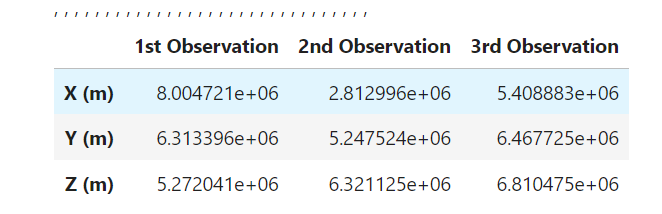# IodGooging. Orbit got from three angular observations

Hello everyone!!

I’ve been trying to implement IodGooding (specifically Orbit got from three angular observations!!) recently, but I’ve got super unsuccessful result for it.

I used real observations of GSS:

``````LAT = 43.05722 # North
LON = 76.971667 # West
ALT = 2735.0 # meters

t1 = Time('2023-06-09T17:04:59.10', format='isot', scale='utc')
t2 = Time('2023-06-09T17:10:50.66', format='isot', scale='utc')
t3 = Time('2023-06-09T17:16:21.09', format='isot', scale='utc')

RA = np.array([(15*(16+5/60+51.20/3600)),
(15*(16+11/60+43.73/3600)),
(15*(16+17/60+15.1/3600))])
DEC = np.array([(-(6+31/60+44.22/3600)),
(-(6+31/60+52.36/3600)),
(-(6+32/60+0.03/3600))])
``````

or data with a time days apart, thus nRev is more than 0 (let’s consider it as 2nd case):

``````
t1 = Time('2019-06-22T15:57:15.87', format='isot', scale='utc')
t2 = Time('2019-06-23T15:57:16.87', format='isot', scale='utc')
t3 = Time('2019-06-24T17:01:04.87', format='isot', scale='utc')

RA = np.array([(15*(15+54/60+36.81/3600)),
(15*(15+58/60+33.43/3600)),
(15*(17+0/60+59.51/3600))])
DEC = np.array([(-(6+2/60+0.16/3600)),
(-(6+1/60+59.38/3600)),
(-(6+32/60+53.21/3600))])
``````

For calculating position I used IOD with Gauss Method.ipynb.

``````r1_vec = position
r2_vec = position
r3_vec = position
r1=(np.dot(r1_vec,r1_vec))**0.5
r2=(np.dot(r2_vec,r2_vec))**0.5
r3=(np.dot(r3_vec,r3_vec))**0.5

# Initialization of Gooding IOD Method
gooding = IodGooding(Mu)

ITRF = FramesFactory.getITRF(IERSConventions.IERS_2010, True)  # Define ECEF reference frame.
earth = OneAxisEllipsoid(Re, f, ITRF)

# Frame of the ground station is given as Topocentric Frame.
station_coord = GeodeticPoint(LAT, LON, ALT)
station_frame = TopocentricFrame(earth, station_coord, "N42")

# Create the ground station.
ground_station = GroundStation(station_frame)

# Create the observable satellite object, name it 1 as default
obs_sat = ObservableSatellite(1)

sigma  = [1.0, 1.0]
baseW  = [1.0, 1.0]

nRev = int(t3.jd - t1.jd)

``````

In 1st case I’ve got next result:

``````GIBBS Kepler elements:
Keplerian parameters: {a: 4.240057888814118E7; e: 0.004565616165528337; i: 0.09156350509937108; pa: 169.65363782985855; raan: 91.0134171094278; v: -18.903633576322076;}

GOODING Kepler elements:
Keplerian parameters: {a: 6.4562346547382206E7; e: 0.2776002076596247; i: 12.165627396844142; pa: -100.77301045351494; raan: -27.59808364817013; v: 11.47979431436121;}
``````

It’s clear, that somethings works not so well with IodGooding. Then I thought that the reason may hide in input data itself. So i tried to run the 2nd case, but it didn’t work, giving out NaN for every element.

I’ll be glad for every thought, that you may have on reason of that strange behavior of IodGooding. Thank you in advance!!

Hi @daiana

GeodeticPoint class uses SI units. Could you try to convert your LAT and LON in radians using FastMath.toRadians()?

Best regards,
Bryan

Thank you, Bryan!! I really haven’t noticed missing converting latitude and longitude, but i still got not good result with fixing this mistake:

``````GOODING Kepler elements:
Keplerian parameters: {a: 6.993022900990523E7; e: 0.3347392113087113; i: 0.5890569678045681; pa: -108.07132218757638; raan: -12.643296530529136; v: 2.5872234997974974;}
``````

Hello @daiana,

Could you give us the values of vectors r1 and r3 you are using please ? There are 2 cases with the reference you gave :or

To be sure to reproduce the issue with the same valuesHello again,

I understood you used the Gauss method to determine the position vectors r1, r2 and r3 :

Vector3D r1 = new Vector3D(-2.0921302099117476E7, -3.6670713616811395E7, 34465.7831621021);
Vector3D r2 = new Vector3D(-1.99735595868321E7, -3.719354402143339E7, 32965.932702185586);
Vector3D r3 = new Vector3D(-1.90708500448641E7, -3.766276304220245E7, 31536.539604075253);

To be noted that the IodGauss method was implemented recently but not released in the current version of Orekit (current 11.3.3, will be released in 12.0).
So I was able to run the different iod methods (Gauss, Gibbs, Lambert, Laplace and Gooding). with the mentionned above position vectors, to build Radec or Position depending on the iod method, the results :

for IodGauss :

• Keplerian parameters: {a: 4.238973759735789E7; e: 0.004324854642335683; i: 0.09157707700428211; pa: 170.7261459980965; raan: 91.00885483327102; v: -19.97157952224498;}

For IodGibbs :

• Keplerian parameters: {a: 4.240057861236801E7; e: 0.0045648965960561755; i: 0.09157707700348447; pa: 169.63021824545928; raan: 91.00885483355044; v: -18.8756517698872;}

For IodGooding :

• Keplerian parameters: {a: 6.993021221010809E7; e: 0.3347390725866758; i: 0.5890565053278204; pa: -108.07120996868652; raan: -12.64337508041537; v: 2.587189785272028;}

For IodLambert :

• Keplerian parameters: {a: 4.238973728430637E7; e: 0.004325701772699008; i: 0.09157707701680115; pa: 170.75757119774016; raan: 91.00885482888536; v: -21.471994338554737;}

For IodLaplace :

• Keplerian parameters: {a: 4.239449499114495E7; e: 0.004440880914435505; i: 0.09000173164942997; pa: 173.17029056487831; raan: 91.20190581104737; v: -22.60868469094336;}

So i have reproduced your problem and indeed it seems there is a problem with IodGooding, I will go deeper in the code to try to find it.

Hi there,

Gooding takes the number of revolution as an input. What did you guys use? Can you try other values?

Cheers,
Romain

Hello @Serrof,

I tested with different nRev and it gives the same result. the Nrev is used in IodGooding with a call to solveLambertPb, which is the core of IodLambert, and i have good results with Lambert when using nRev = 0, so I think it does not come from here.

I suggest to open an issue to study specifically the code of IodGooding, based on the paper “A NEW PROCEDURE FOR THE SOLUTION OF THE CLASSICAL
PROBLEM OF MINIMAL ORBIT DETERMINATION FROM THREE
LINES OF SIGHT”, with a fortran algorithm as a source for orekit code.

It seems also that we could improve the IodGooding to N measurements as well as optimise it : “Modifications to the Gooding Algorithm for Angles-Only Initial OrbitDetermination”

My code here to reproduce the problem in java (delete IodGauss call if not on dev version) :
IodGooding_bug.txt (4.4 KB)

Thank you, @jasquier, for your help. Are you going to open an issue with IodGooding method problem, or should I do this??

Have you tried to run the 2nd case, by the way?? If you have, what was the result, and was it affected by changing nRev parameter??

Hi @daiana,

Thank you for reporting the problem!
Please open the issue since you are the original reporter.

Cheers,
Maxime

1 Like

Gooding method looks to be less accurate with big time interval for GEO test cases. At the opposite of the other methods for which the error increase gently for the orbit shape . Here your observation interval is 48H which is big for IOD.